### 10.5.1.2 Computing a saddle point

The security plan for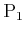constitutes a valid solution to the game under the alternating play model.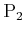has only to choose an optimal response to the plan ofat each stage. Under the stage-by-stage model, the solution'' concept is a saddle point, which occurs when the upper and lower values coincide. The procedure just described could be used to determine the value and corresponding plans; however, what happens when the values do not coincide? In this case, randomized security plans should be developed for the players. As in the case of a single-stage game, a randomized upper value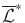and a randomized lower value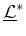are obtained. In the space of randomized plans, it turns out that a saddle point always exists. This implies that the game always has a randomized value,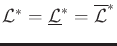. This saddle point can be computed from the bottom up, in a manner similar to the method just used to compute security plans.

Return to the example in Figure 10.13. This game actually has a deterministic saddle point, as indicated previously. It still, however, serves as a useful illustration of the method because any deterministic plan can once again be interpreted as a special case of a randomized plan (all of the probability mass is placed on a single action). Consider the bottom four subtrees of Figure 10.13, which are obtained by using only the last two levels of decision vertices. In each case,andmust act in parallel to end the sequential game. Each subtree can be considered as a matrix game because the costs are immediately obtained after the two players act.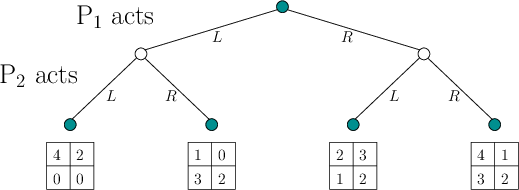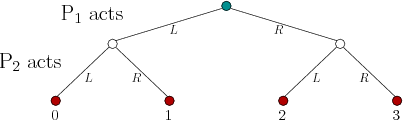This leads to an alternative way to depict the game in Figure 10.13, which is shown in Figure 10.17. The bottom two layers of decision vertices are replaced by matrix games. Now compute the randomized value for each game and place it at the corresponding leaf vertex, as shown in Figure 10.18. In the example, there are only two layers of decision vertices remaining. This can be represented as the game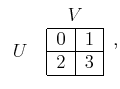(10.104)

which has a value ofand occurs ifapplies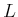andapplies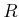. Thus, the solution to the original sequential game has been determined by solving matrix games as an alternative to the method applied to obtain the security plans. The benefit of the new method is that if any matrix does not have a deterministic saddle point, its randomized value can instead be computed. A randomized strategy must be played by the players if the corresponding decision vertex is reached during execution.

Steven M LaValle 2020-08-14